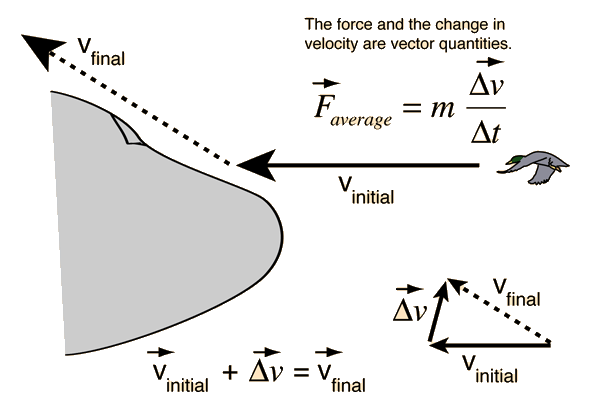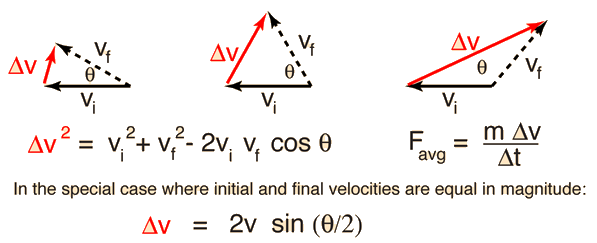# Force of Glancing Blow

The force of collision between an airliner and a duck can be diminished greatly if the duck bounces off. The impact force is given by:The impact force is reduced by the fact that the change in velocity is much less for the glancing blow. The vector addition which gives the final velocity shows that for a small angle deflection, the velocity change is small. The change in velocity may be calculated using the law of cosines. In the elastic case where initial and final speeds are equal, the half-angle formula may be used to obtain a simplified expression.Note that the impact force is not always less for a glancing blow. If the deflection angle is greater than 60 degrees, the impact force will be greater than that for a head-on inelastic collision.

The calculation below explores variations in deflection angle for the problem in which the airliner travels at 600 mi/hr and collides with a 1 pound, 1 foot long duck. This calculation assumes a collision time of 1/880 sec for consistency with head-on case. It is assumed that the collision is elastic, so that the duck is deflected without changing its speed. If the duck is deflected through an angle of degrees, then the average impact force would be tons, compared to an impact force of 12.1 tons for a head-on, inelastic collision.

### Calculate for inelastic case

 Minimizing impact force Airplane and duck collision Big truck, small truck collision
Index

Collision concepts
HyperPhysics***** Mechanics ***** Newton's laws Go Back